# 数据挖掘入门系列教程（七点五）之神经网络介绍

2019/04/10 10:10

[TOC]

## 数据挖掘入门系列教程（七点五）之神经网络介绍

### 简介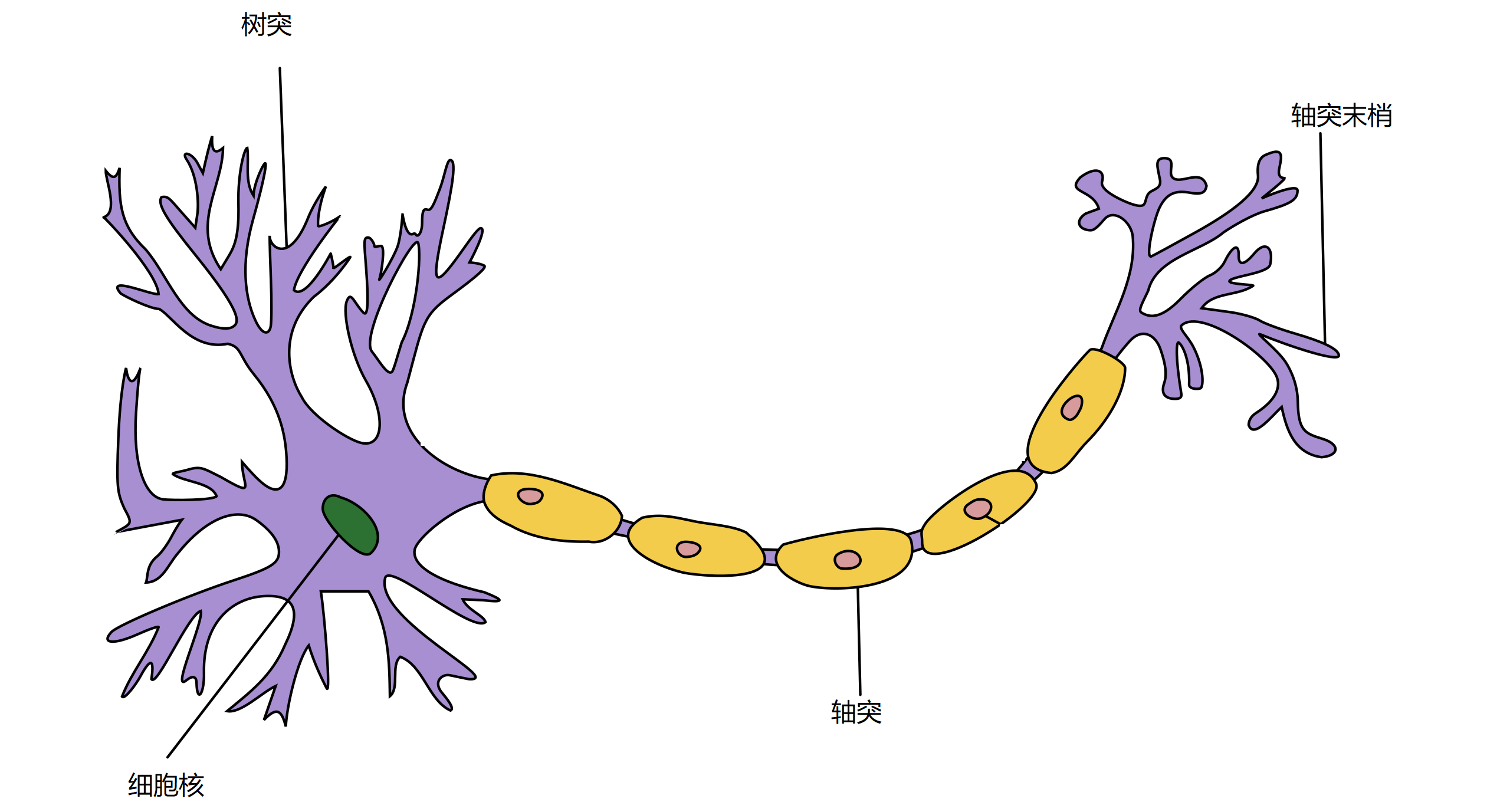### M-P 模型

M-P，emm，就是上面两位科学家的命名（McCulloch-Pitts）。M-P模型如下：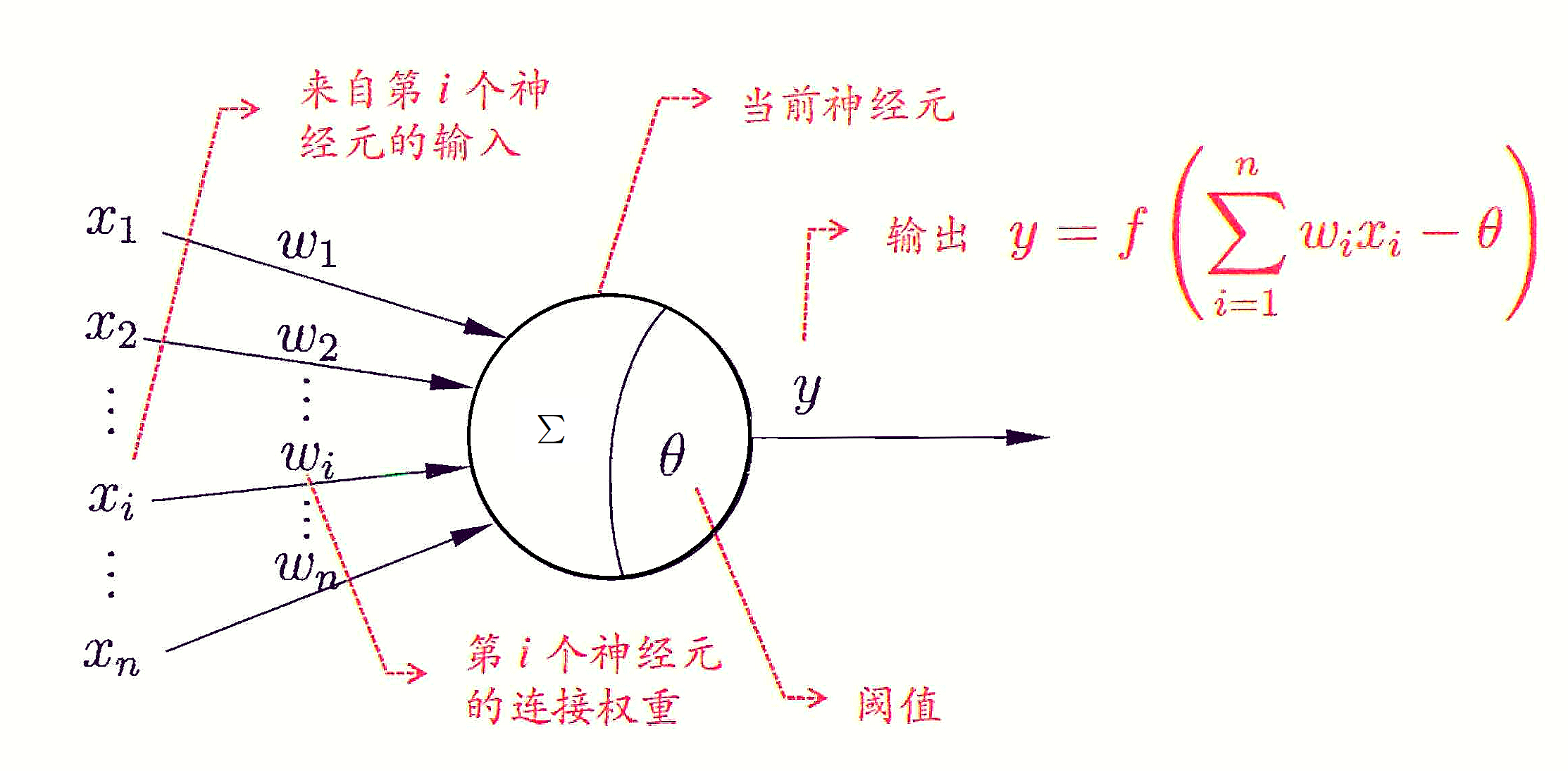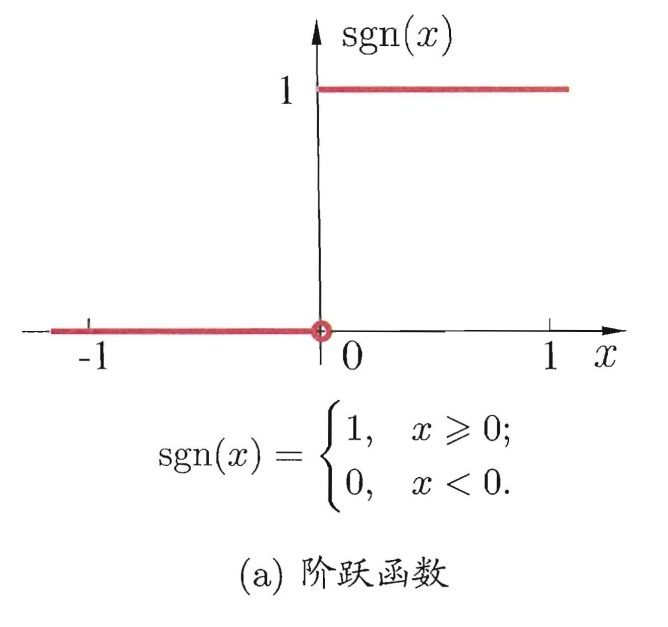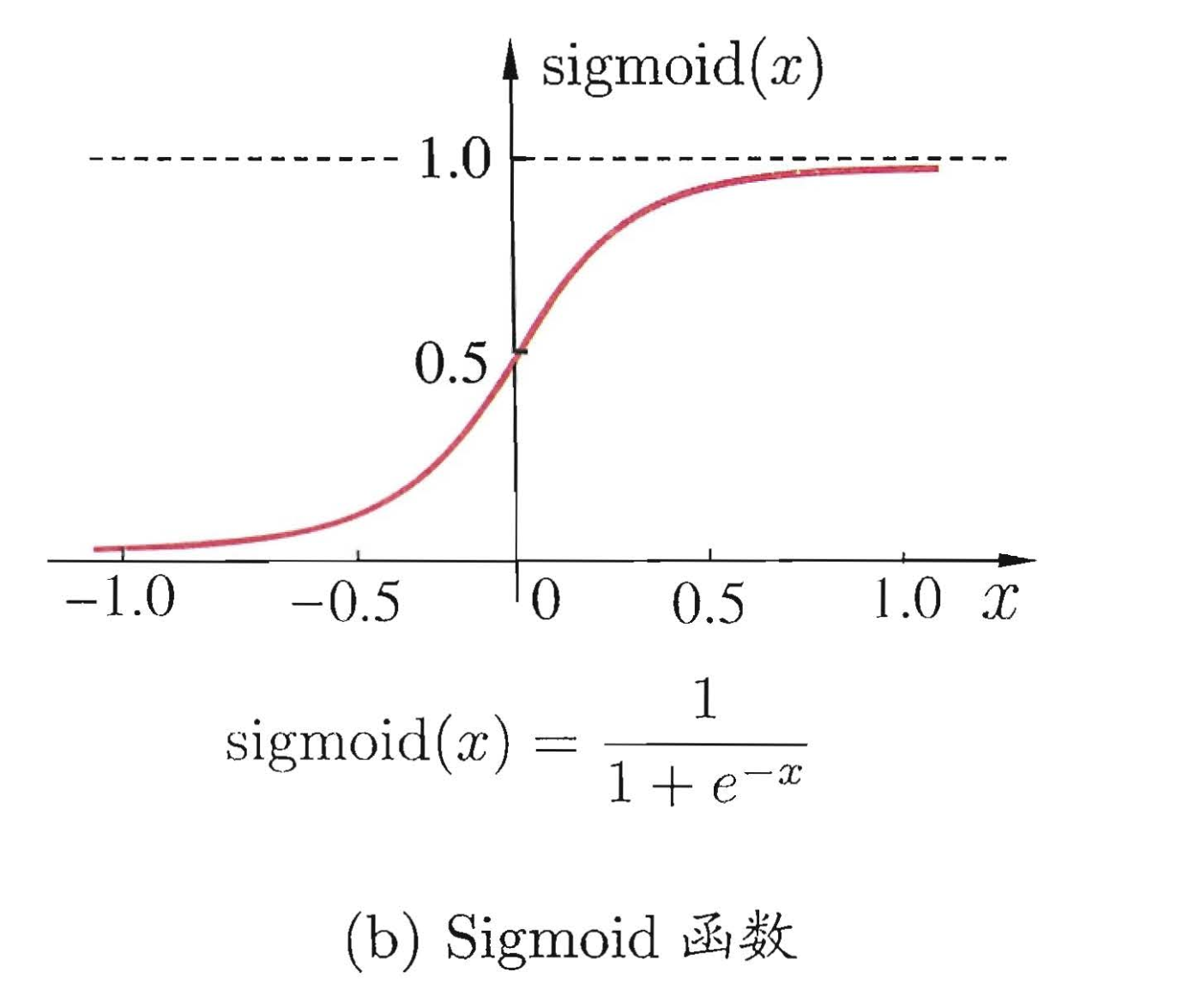### 感知机（两层神经网络）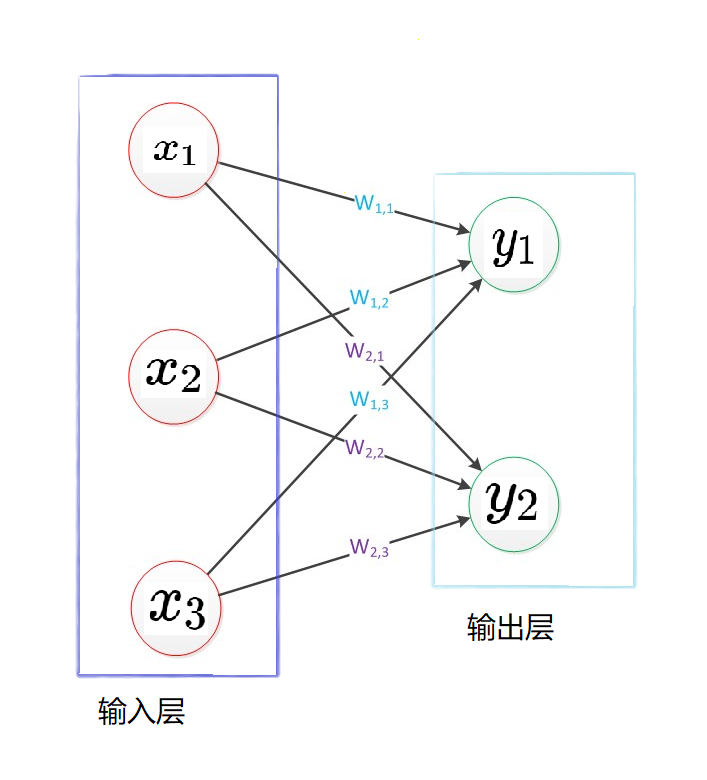#### 权重学习

\begin{aligned} &w_{i} \leftarrow w_{i}+\Delta w_{i}\ &\Delta w_{i}=\eta(y-\hat{y}) x_{i} \end{aligned}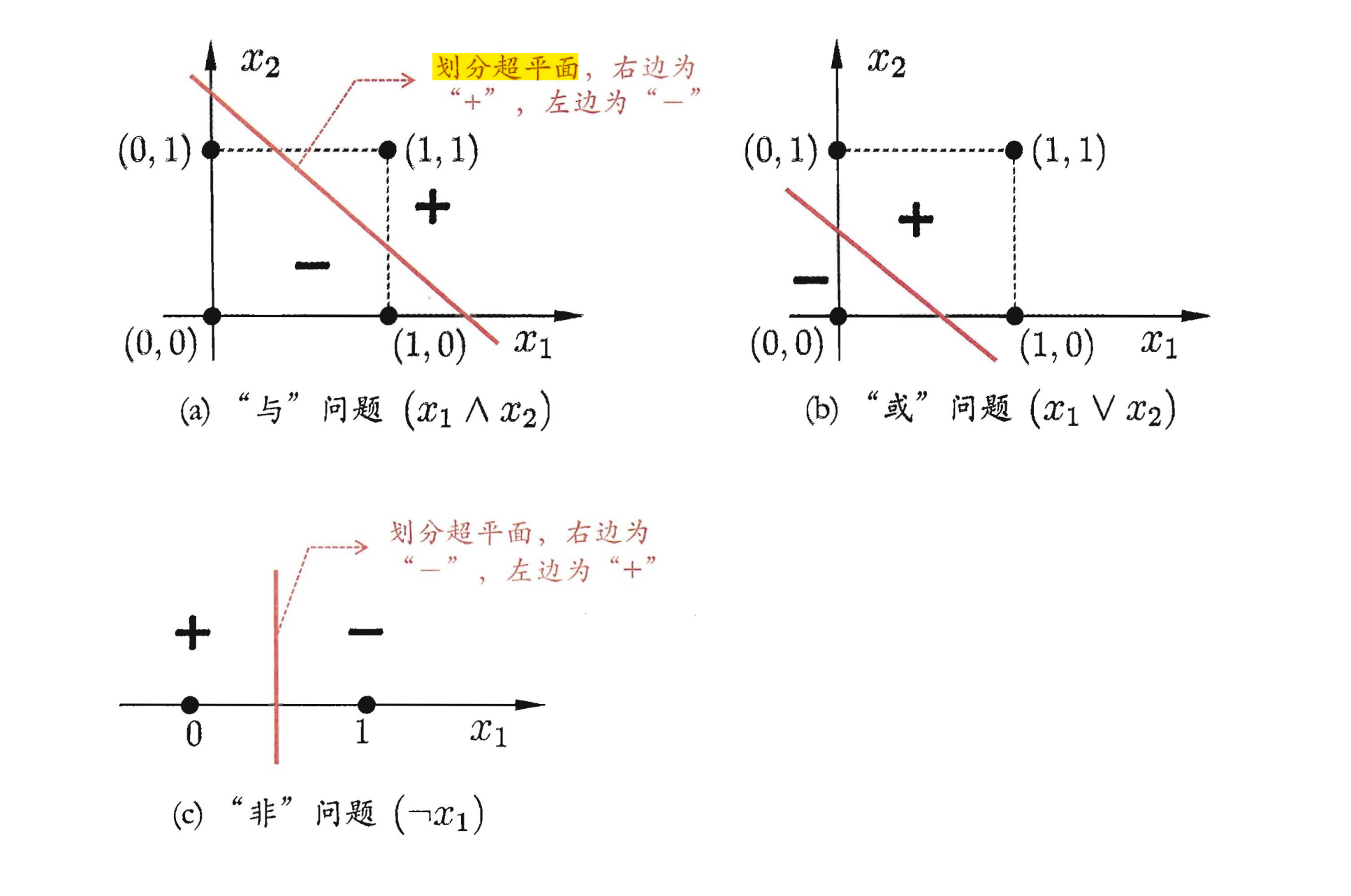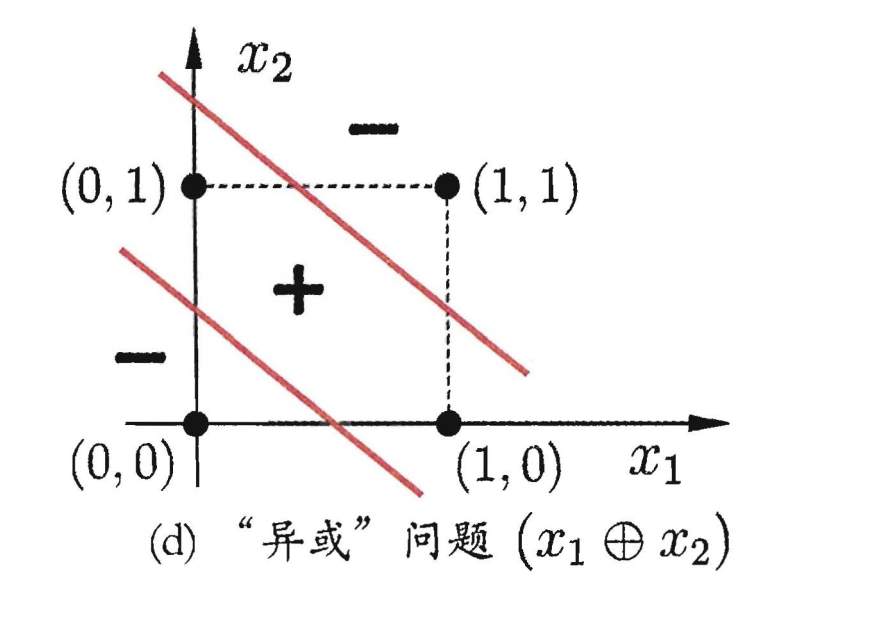### 多层神经网络（多层感知机）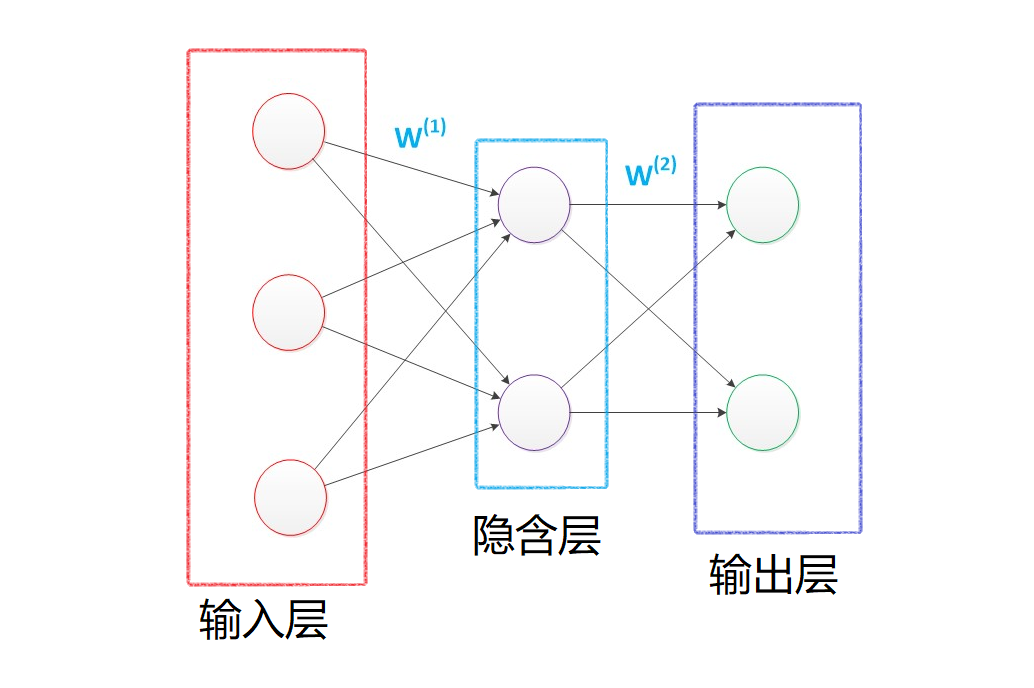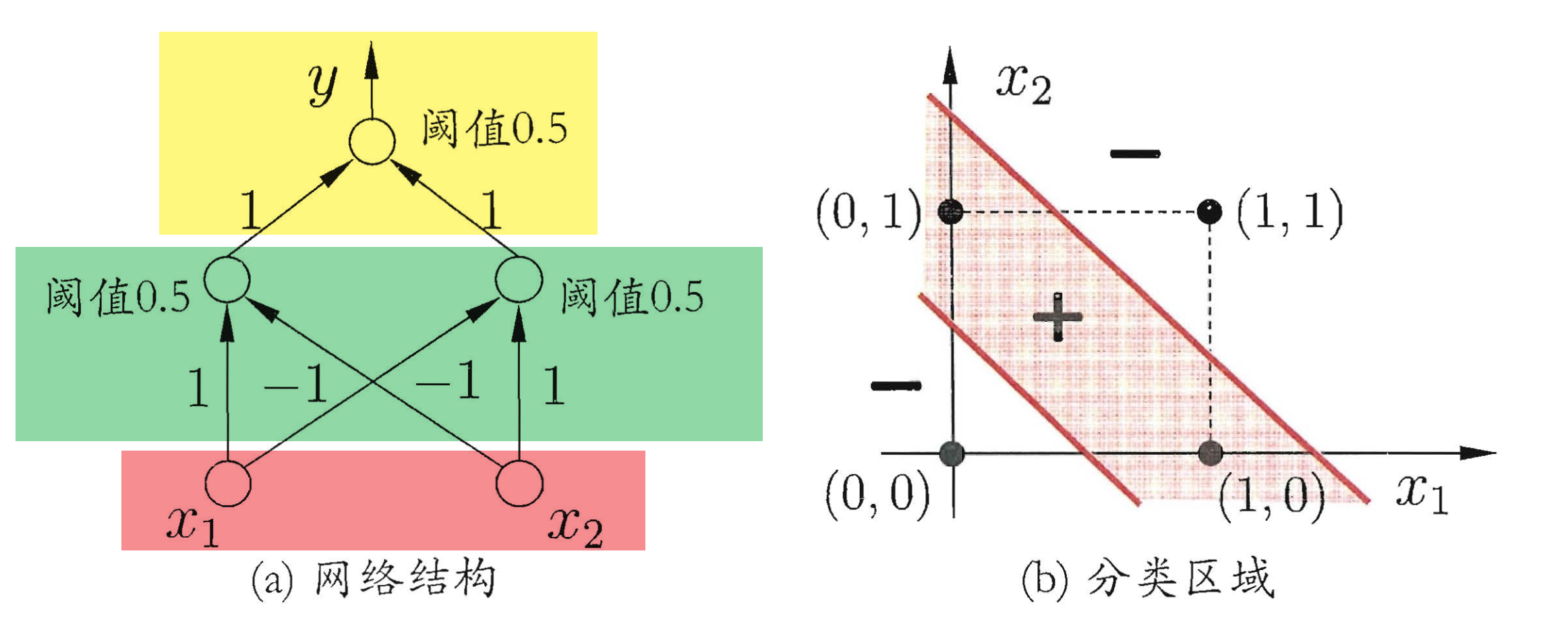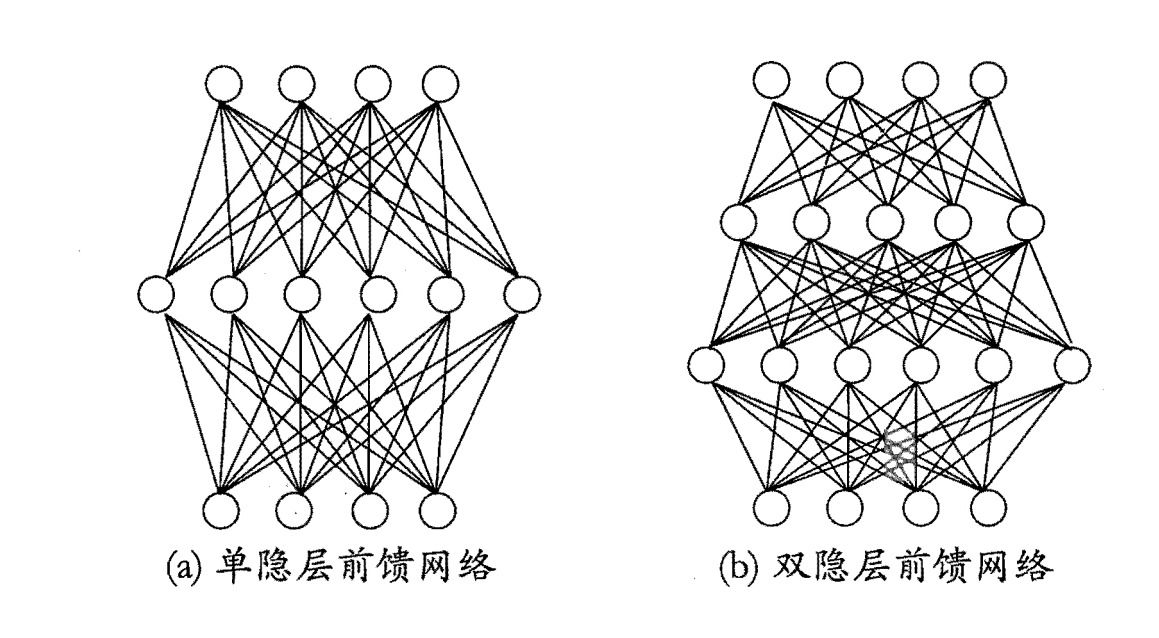#### 连接权学习——BP算法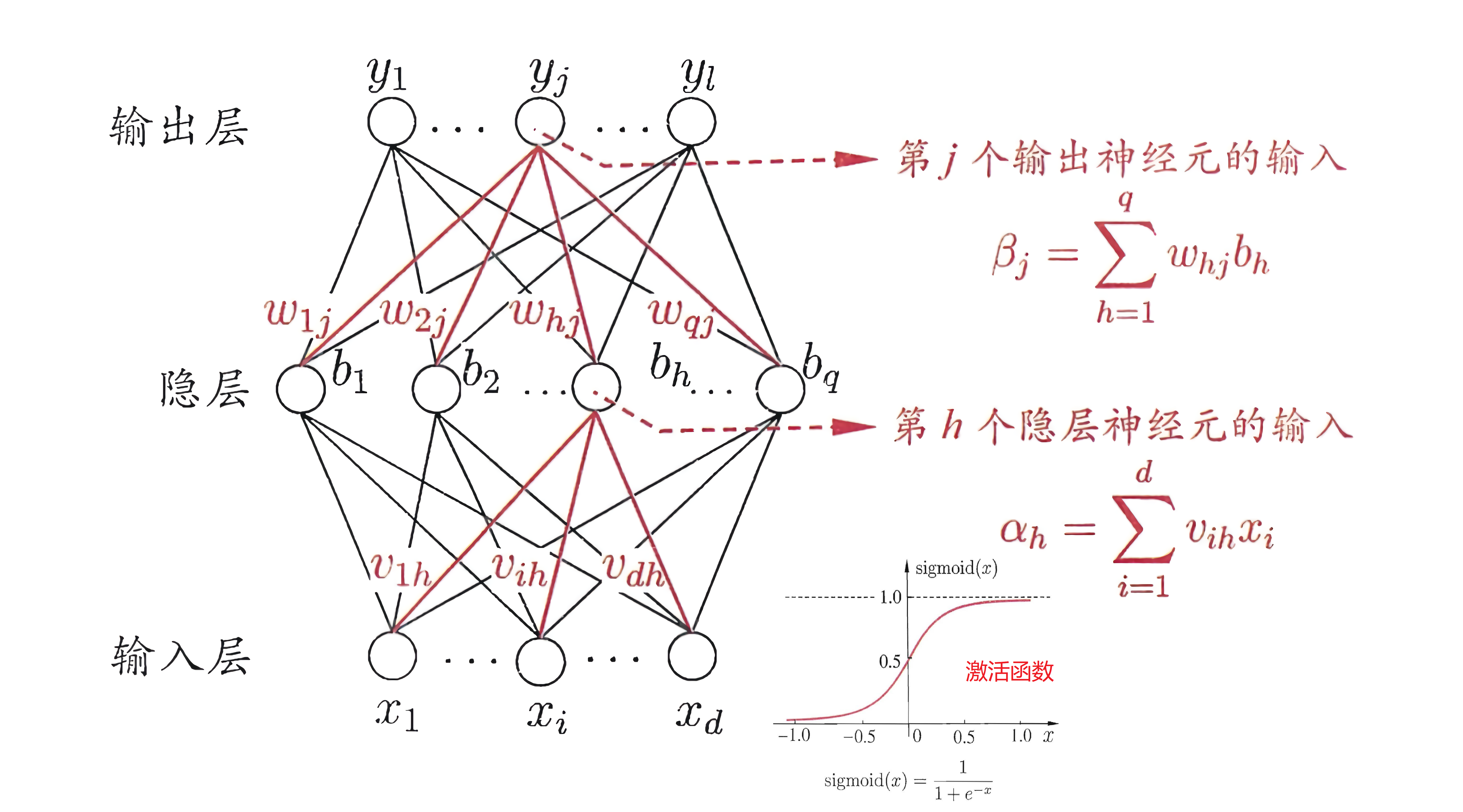$\hat{y}{j}^{k}=f\left(\beta{j}-\theta_{j}\right)$

$E_{k}=\frac{1}{2} \sum_{j=1}^{l}\left(\hat{y}{j}^{k}-y{j}^{k}\right)^{2}$

$v \leftarrow v+\Delta v$

BP算法基于梯度下降策略，以目标的负梯度方向对参数进行调整。对于均方误差，给定学习率$\eta$，有： $\begin{equation}\Delta w_{h j}=-\eta \frac{\partial E_{k}}{\partial w_{h j}}\end{equation}$ 对于$w_{hj}$，它先影响第$j$个输出层神经元的输入$\beta_{j}$，然后再影响输出值$\hat{y}{j}^{k}$，最后影响$E_k$，根据导数的规律，有： $\begin{equation} \frac{\partial E{k}}{\partial w_{h j}}=\frac{\partial E_{k}}{\partial \hat{y}{j}^{k}} \cdot \frac{\partial \hat{y}{j}^{k}}{\partial \beta_{j}} \cdot \frac{\partial \beta_{j}}{\partial w_{h j}} \ \end{equation}$ 然后对于$\beta_{j}$有： $\frac{\partial \beta_{j}}{\partial w_{h j}}=b_{h}$ 对于对于$Sigmoid$函数有： $f^{\prime}(x)=f(x)(1-f(x))$

\begin{equation}\begin{aligned} &\because \hat{y}{j}^{k}=f\left(\beta{j}-\theta_{j}\right)\ &\because E_{k}=\frac{1}{2} \sum_{j=1}^{l}\left(\hat{y}{j}^{k}-y{j}^{k}\right)^{2} \ \therefore g_{j} &=-\frac{\partial E_{k}}{\partial \hat{y}{j}^{k}} \cdot \frac{\partial \hat{y}{j}^{k}}{\partial \beta_{j}} \ &=-\left(\hat{y}{j}^{k}-y{j}^{k}\right) f^{\prime}\left(\beta_{j}-\theta_{j}\right) \ &=(y_{j}^{k}-\hat{y}{j}^{k})\hat{y}{j}^{k}(1-\hat{y}_{j}^{k}) \end{aligned}\end{equation}

\begin{equation}\begin{aligned} \frac{\partial E_{k}}{\partial \gamma_{h}} &=\sum_{j=1}^{l} \frac{\partial E_{k}}{\partial \hat{y}{j}^{k}} \cdot \frac{\partial \hat{y}{j}^{k}}{\partial \beta_{j}} \cdot \frac{\partial \beta_{j}}{\partial b_{h}} \cdot \frac{\partial b_{h}}{\partial \gamma_{h}} \ &=\sum_{j=1}^{l} \frac{\partial E_{k}}{\partial \hat{y}{j}^{k}} \cdot \frac{\partial \hat{y}{j}^{k}}{\partial \beta_{j}} \cdot \frac{\partial \beta_{j}}{\partial b_{h}} \cdot f^{\prime}\left(\alpha_{h}-\gamma_{h}\right) \cdot(-1) \ &=-\sum_{j=1}^{l} \sum_{\partial j}^{\partial E_{k}} \frac{\partial \hat{y}{j}^{k}}{\mathbb{G}{j}} \cdot w_{h j} \cdot f^{\prime}\left(\alpha_{h}-\gamma_{h}\right) \ &=-\sum_{j=1}^{l} \frac{\partial E_{k}}{\partial \hat{y}{j}^{k}} \cdot \frac{\partial \hat{y}{j}^{k}}{\partial \beta_{j}} \cdot w_{h j} \cdot b_{h}\left(1-b_{h}\right) \ &=\sum_{j=1}^{l} g_{j} \cdot w_{h j} \cdot b_{h}\left(1-b_{h}\right) \ &=e_{h} \end{aligned}\end{equation} 因此： $\begin{equation}\Delta \gamma_{h}=-\eta \frac{\partial E_{k}}{\partial \gamma_{h}}=-\eta e_{h}\end{equation}$ 综上可得： $\begin{equation}\begin{array}{l} \Delta w_{h j}=\eta g_{j} b_{h} \ \Delta \theta_{j}=-\eta g_{j} \ \Delta v_{i h}=\eta e_{h} x_{i} \ \Delta \gamma_{h}=-\eta e_{h} \ 学习率\eta不一定相等 \end{array}\end{equation}$ 其中： \begin{equation}\begin{aligned} &g_{j}=\left(y_{j}^{k}-\hat{y}{j}^{k}\right) \hat{y}{j}^{k}\left(1-\hat{y}{j}^{k}\right)\ &e{h}=\sum_{j=1}^{l} g_{j} \cdot w_{h j} \cdot b_{h}\left(1-b_{h}\right)\ \end{aligned}\end{equation} 算法的流程如下：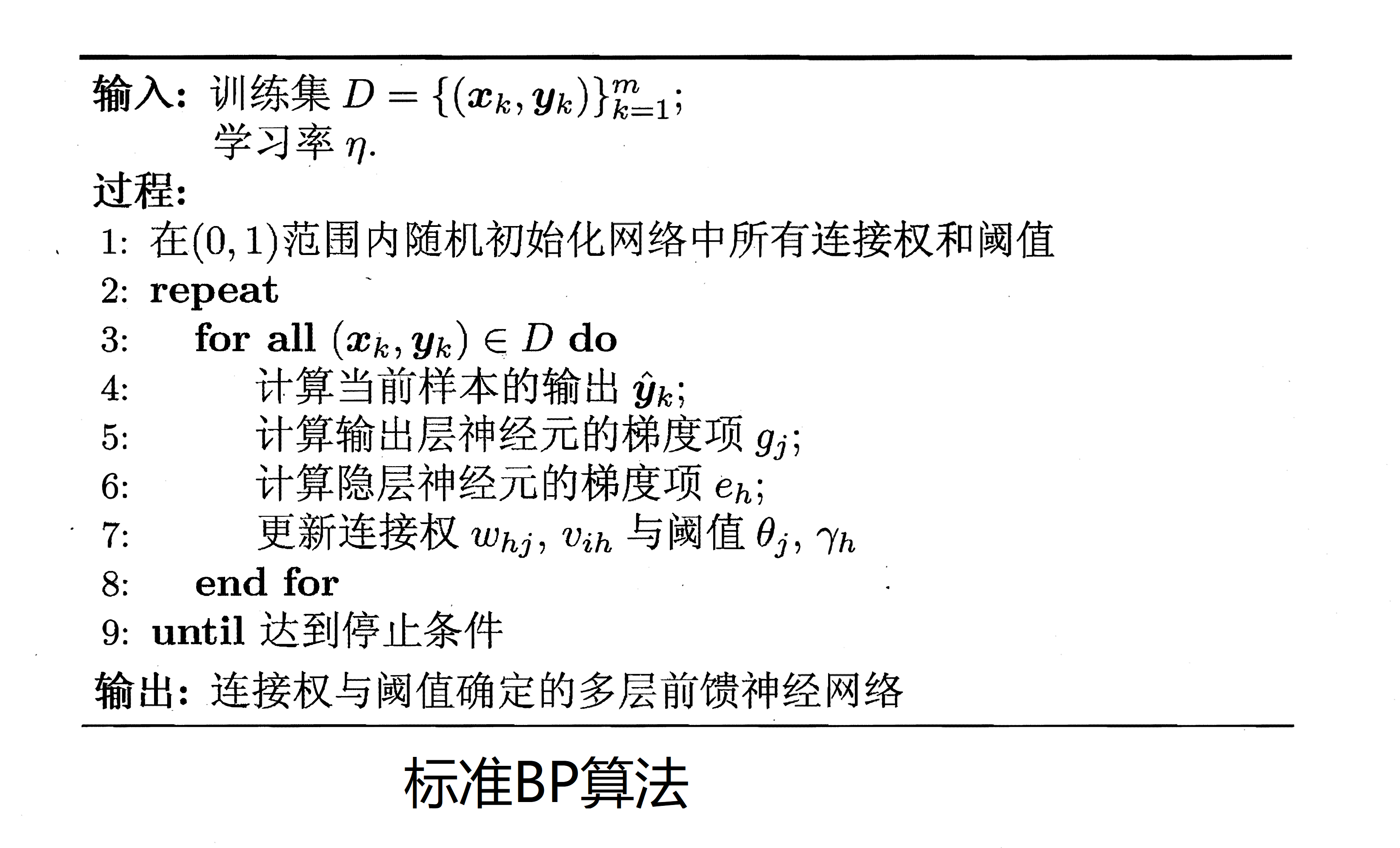#### 防止过拟合

• 早停（early stopping）

将数据集分成训练机和测试集，训练集用来更新连接权和阈值，测试集用来验证累计误差。若训练集误差降低但是测试集误差升高，则停止训练。

• 正则化（regularization）

正则化的思想就是在误差目标函数中增加一个用于描述网络复杂度的部分。这里添加连接权和阈值的平方和。此时误差目标函数就变成了： $\begin{equation} E=\lambda \frac{1}{m} \sum_{k=1}^{m} E_{k}+(1-\lambda) \sum_{i} w_{i}^{2} \ \lambda \in(0,1) \end{equation}$

增加连接权与闵值平方和这一项后，训练过程将会偏好比较小的连接权和阈值，使网络输出更加"光滑"，从而对过拟合有所缓解.

### 局部最小和全局最小

• 局部最优
• 全局最优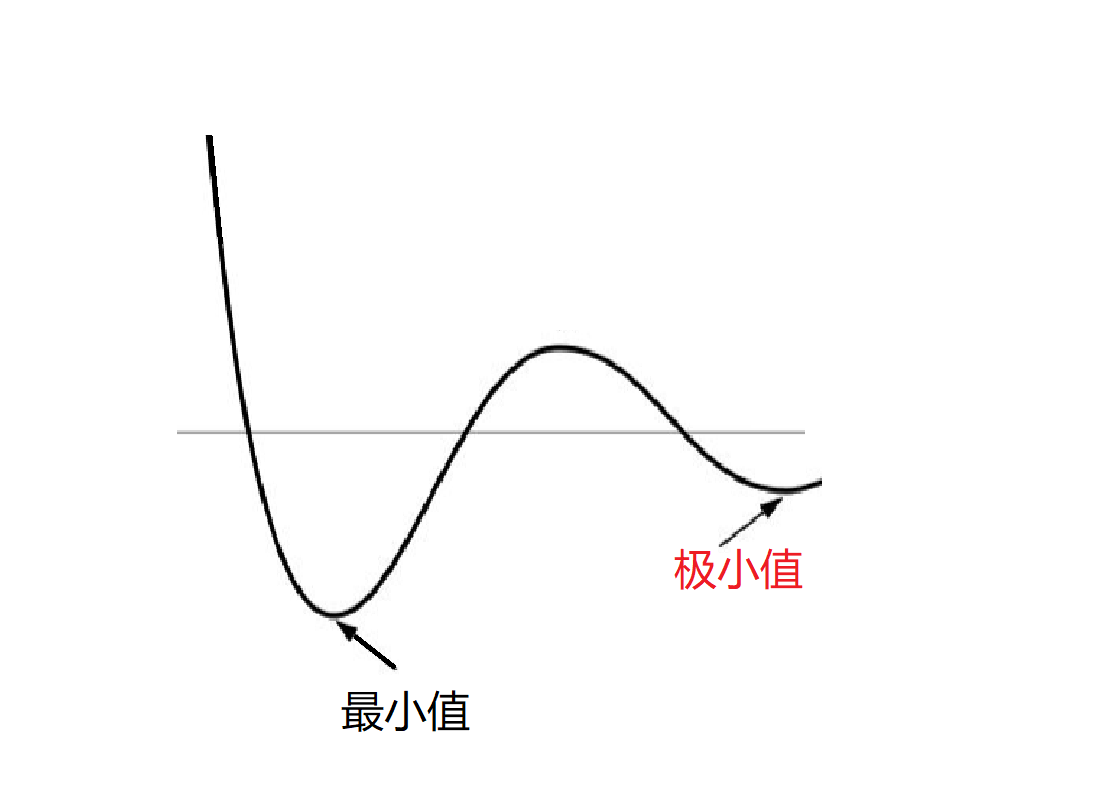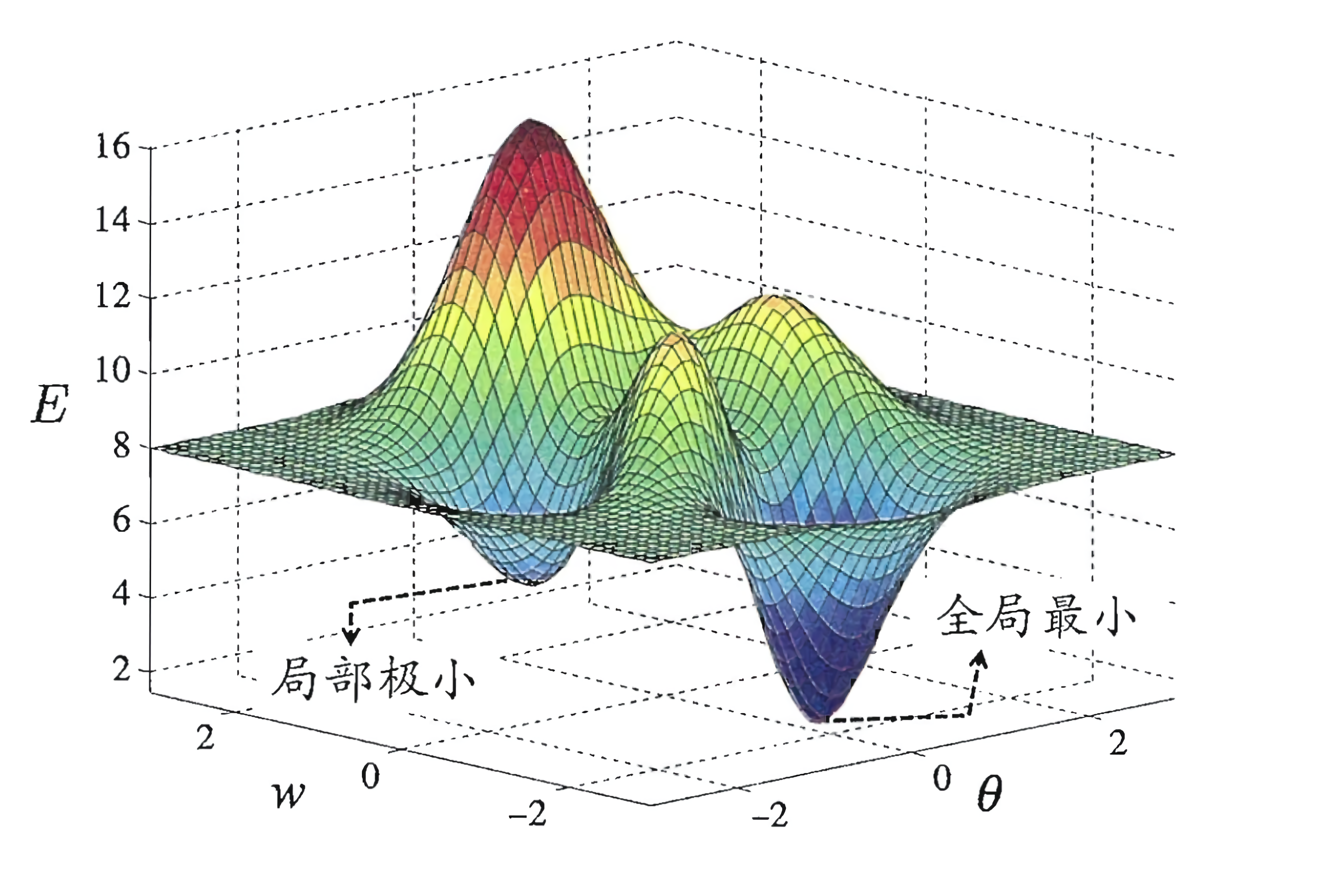• 以多组不同参数值初始化多个神经网络，按标准方法训练后，取其中误差最小的解作为最终参数。这相当于从多个不同的初始点开始搜索,这样就可能陷入不同的局部极小，从中进行选择有可能获得更接近全局最小的结果。简单点来说就是从多个较小值中取出一个最小值。
• 使用“模拟退火”(simulated annealing)技术。模拟退火在每一步都以一定的概率接受比当前解更差的结果，从而有助于“跳出”局部极小。在每步迭代过程中，接受“次优解”的概率要随着时间的推移而逐渐降低，从而保证算法稳定。
• 使用随机梯度下降.与标准梯度下降法精确计算梯度不同，随机梯度下降法在计算梯度时加入了随机因素。于是，即便陷入局部极小点，它计算出的梯度仍可能不为零,这样就有机会跳出局部极小继续搜索。
• 遗传算法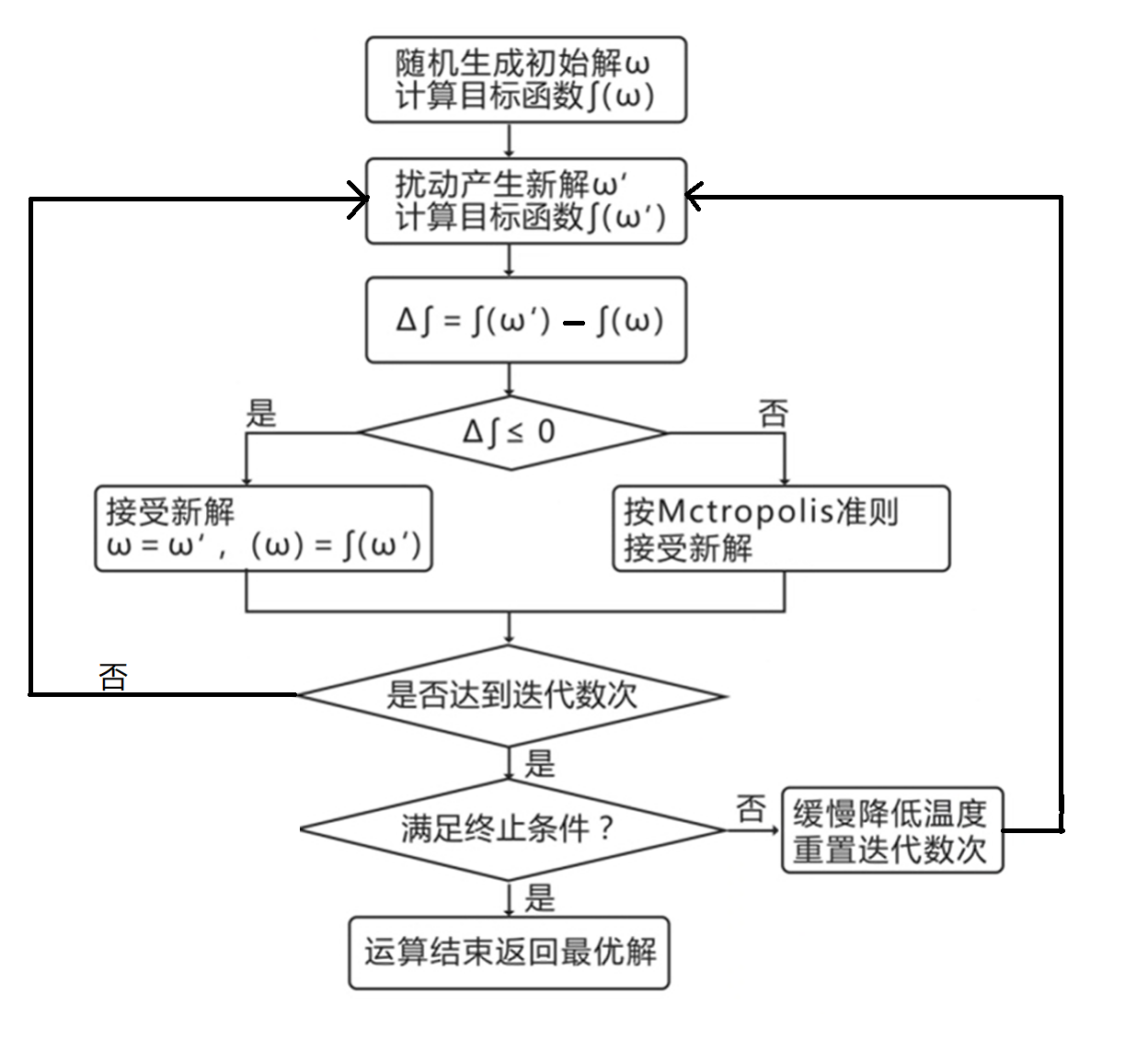### 总结

OK，神经网络就暂时介绍到这里，至于深度学习这些内容，以后再做介绍，毕竟代码不是一天写成的。这一篇博客主要是为下一篇博客的使用神经网络识别验证码做铺垫。因为在《Python数据挖掘入门与实践》中并没有对神经网络做介绍。

#### 参考

1. 《西瓜书》——周志华
2. 人工神经网络——维基百科
3. 南瓜书
4. 为什么神经网络能以任意精度拟合任意复杂度的函数？
5. 模拟退火算法
6. 神经网络浅讲：从神经元到深度学习### 作者的其它热门文章

0
0 收藏

0 评论
0 收藏
0